cancel
Showing results for
Did you mean:## Decoding Direct Query in Power BI Part 2: Date Difference Across in Direct Query: Oracle/SQL Server

Power BI Direct Query allows users to create a direct connection with the database and work without importing the data into Power BI. There are a lot of questions about what works and what does not. In the second article of the series, Decoding Direct Query, we will explore how to determine the date difference across two tables. The direct query does not support the new column, therefore, taking the difference between the dates of the same table also required a similar approach.

Setup:

Last time, we used SQL Server Express Edition, but this time we decided to experiment with Oracle. Oracle 18c Express edition is available from Oracle. This edition is also supported by Direct Query. And we have installed the same. Oracle client installation is also required to access data in Power BI. While installation is easy, but if you forget to give the path for the driver, in the environment path variable of Windows, post-installation then it will not work.

The questions we are going to address:

• Is there any difference between SQL Server and Oracle for the Time intelligence function used in the last article?
• How to get the date difference across two days in two different tables.
• Are date difference formulas similar in SQL Server and Oracle?

Step 1:

Connect Oracle and while connecting choose Direct Query Option.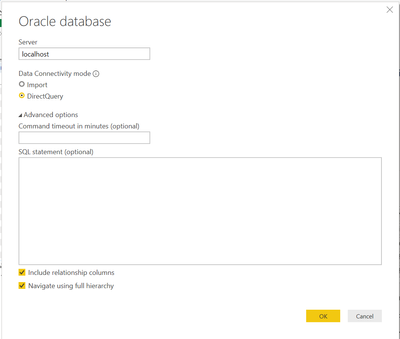Step 2:

Drag all the required tables into Power BI and create the relationship diagram as given below. We have taken two facts and you can see both are connected to the common dimensions. The difference from last time is that we have an additional common Order dimension. To keep the experiment the same, we have added the same dimension to our SQL server pbix. This means both databases and pbix are the same now.

There are a few differences.

• Oracle did not allow us the names like Date and Order as table and column names, so we renamed a few columns. There are ways we can keep those names. But I prefer to change those.
• All table names are in caps in the case of Oracle when we move them to Power BI.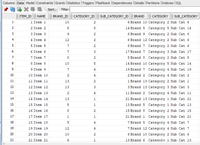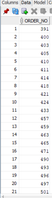SQL ServerStep 3: Mark Timetable

Same as what we did last time. Just right-click on the table in the Visualization tab.Step 4: Change Data Type

Click on any table field/column. You will see column tools as one of the tabs. You have the option to change the data type there. I wanted to make the year as text in date dimension.

Step 5: Change Sort column

Again, under Column Tools you have the option for Sort by column. Use that. We have changed sort for Month Year.

Step 6: Create formulas

Our data do not have Gross Sales or Net Sales as values. So, we need measures. Now, these calculations are needed at the line level. So, we use sumx function as we do not have option to create column.

``````Gross Sales = sumx('ORDERS','ORDERS'[Qty]*'ORDERS'[Price])
Net Sales = sumx('ORDERS',('ORDERS'[Qty]*'ORDERS'[Price])* (100-'ORDERS'[Discount_Percent])/100)
Discount Amount = sumx('ORDERS',('ORDERS'[Qty]*'ORDERS'[Price])*'ORDERS'[Discount_Percent]/100)``````

Step 7: Create Time Intelligence formula

We have created formulas using dates functions like datesytd, datesqtd and datesmtd.

We found no difference in the usages of dates* functions. But Initially, we found Oracle is slow. We created indexes on the Oracle table post that that speed was almost same. The impressive part was Power BI cache.

``````Net Sales LMTD = CALCULATE([Net Sales],DATESMTD(DATEADD(DATEDIM[DDATE],-1,MONTH)) )
Net Sales LQTD = CALCULATE([Net Sales],DATESQTD(DATEADD(DATEDIM[DDATE],-1,QUARTER)))
Net Sales LYMTD = CALCULATE([Net Sales],DATESMTD(DATEADD(DATEDIM[DDATE],-1,YEAR)) )
Net Sales LYQTD = CALCULATE([Net Sales],DATESQTD(DATEADD(DATEDIM[DDATE],-1,YEAR)) )
Net Sales LYTD = CALCULATE([Net Sales],DATESYTD(DATEADD(DATEDIM[DDATE],-1,YEAR)) )
Net Sales MTD = CALCULATE([Net Sales],DATESMTD(DATEDIM[DDATE]))
Net Sales QTD = CALCULATE([Net Sales],DATESQTD(DATEDIM[DDATE]))
Net Sales YTD = CALCULATE([Net Sales],DATESYTD(DATEDIM[DDATE]))
``````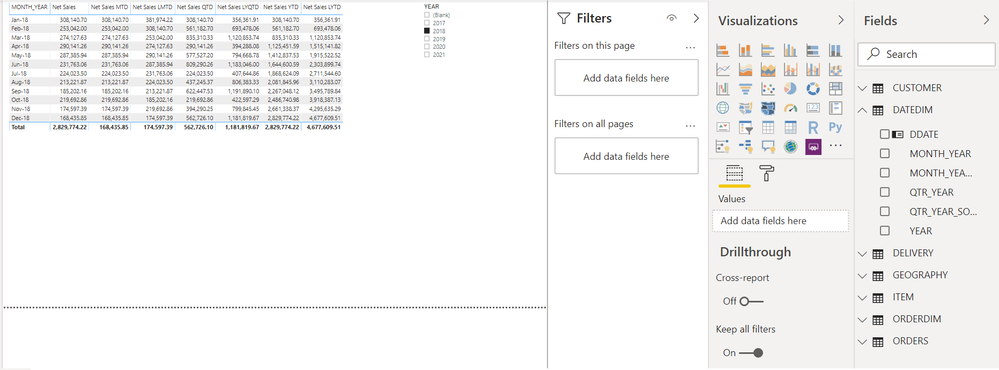Step 8: Create formulas for the date difference.

Time difference using measure requires a context (group by), so we have used order number here from order dimension that is one of the common tables between two. Values and Summarize functions helped us to achieve it.

``````Max Delivery Date = max(DELIVERY[DEILVERY_DATE])
//Oracle
Min Order Date = MIN(ORDERS[SALES_DATE])
Date Diff = AVERAGEX(VALUES(ORDERDIM[ORDER_NO]) ,datediff([Min Order Date],[Max Delivery Date],DAY))
Date Diff 2 = AVERAGEX(SUMMARIZE(ORDERDIM,ORDERDIM[ORDER_NO] ,"_min",[Min Order Date],"_max",[Max Delivery Date]),datediff([_min],[_max],DAY))

//SQL Server
Max Delivery Date = max(DELIVERY[DEILVERY_DATE])
Min Order Date = MIN('order'[SALES_DATE])
Date Diff = AVERAGEX(VALUES(ORDERDIM[ORDER_NO]) ,datediff([Min Order Date],[Max Delivery Date],DAY))
Date Diff 2 = AVERAGEX(SUMMARIZE(ORDERDIM,ORDERDIM[ORDER_NO] ,"_min",[Min Order Date],"_max",[Max Delivery Date]),datediff([_min],[_max],DAY))
``````There is no difference between these formulas between Oracle, SQL Server and import mode.  In Import mode we were able to create a new column across table and were able to get the difference between dates within the table.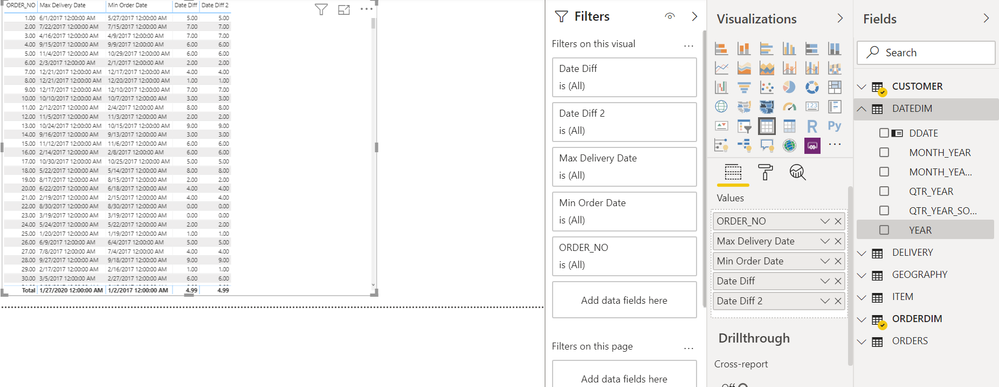In the case of the same table date difference, you do not need a common dimension. You can use primary key of the table.

Do you want us to cover any other topic on Direct Query Mode? Comment and let us know.

This time, I'm not attaching the pbix, as you do not have Database access and it will not work.